# Pie Charts

With the bake sale approaching, Tom asked his mother to help him make apple pie.

He said that if she gave him the recipe, then he would make it.

Mother then drew a circle and divided it into sections that showed the proportion of each ingredient.

This representation is a pie chart, and in this chapter, we will show you examples with an explanation of the pie chart, and pie chart percentages.

Check out the interactive simulations to know more about the lesson and try your hand at solving a few interesting practice questions at the end of the page.

## Lesson Plan

 1 What Are Pie Charts? 2 Solved Examples on Pie Charts 3 Interactive Questions on Pie Charts 4 Challenging Questions on Pie Charts

## What Are Pie Charts?

A pie chart is a type of graph that visually displays data in a circular graph.

Pie charts are one of the most commonly used graphs to represent data using the attributes of circles, spheres, and angular data to represent real-world information.

Pie charts are circlular charts that record discrete data.

Pie represents the whole and the slices represent the parts of the whole.

The value of each sector is to be known, primarily.

The pie chart below shows the ingredients used in a butter cake: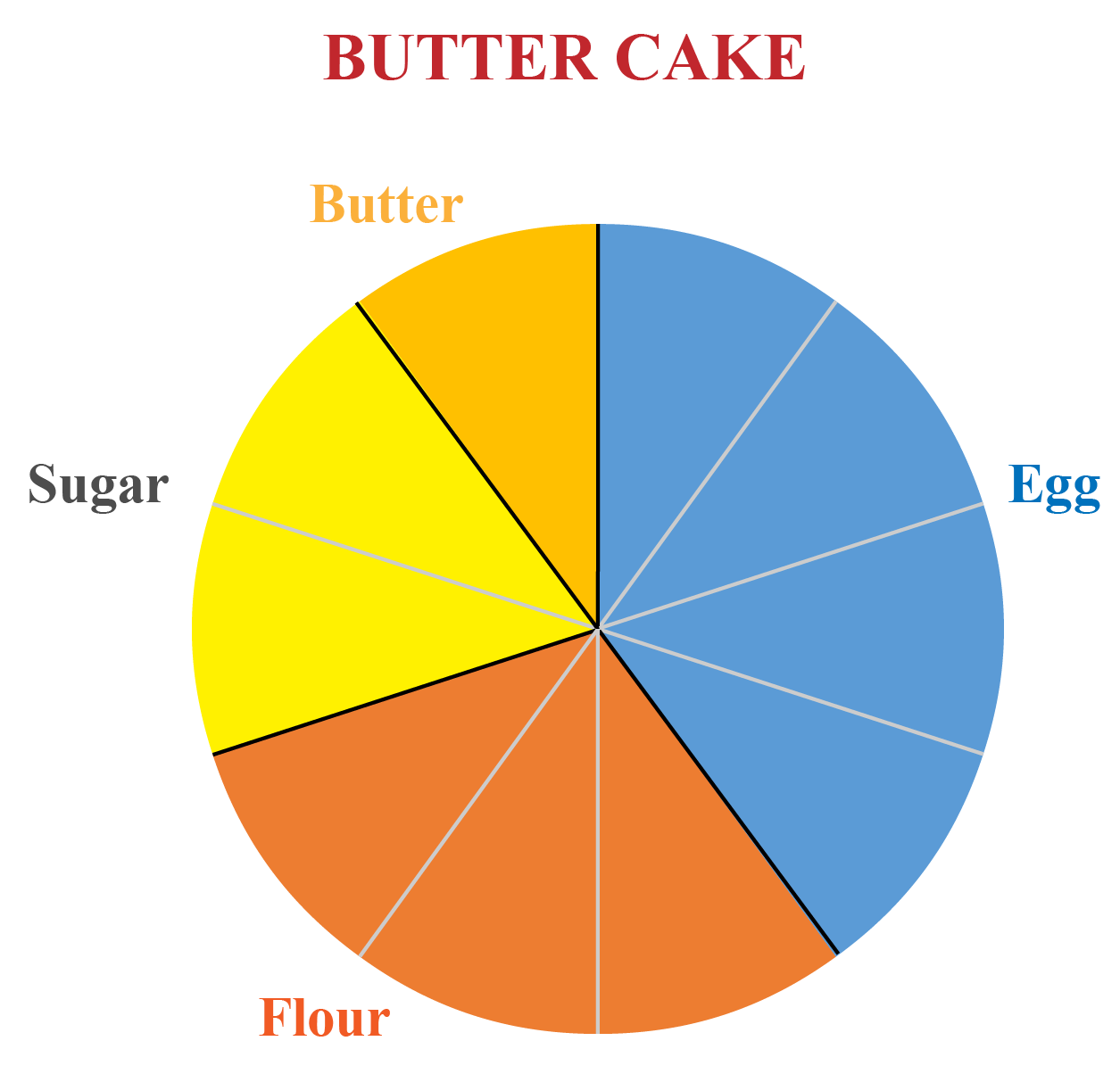The pie is divided into 10 sectors.

Hence each sector's value is $$100\div 10=10$$

Hence this information is interpreted and the table is completed as:

 Ingredients Quantity Flour Sugar Egg Butter 30 20 40 10

## Pie Chart Formula

According to the pie chart formula, the total value of the pie is always $$100 \%$$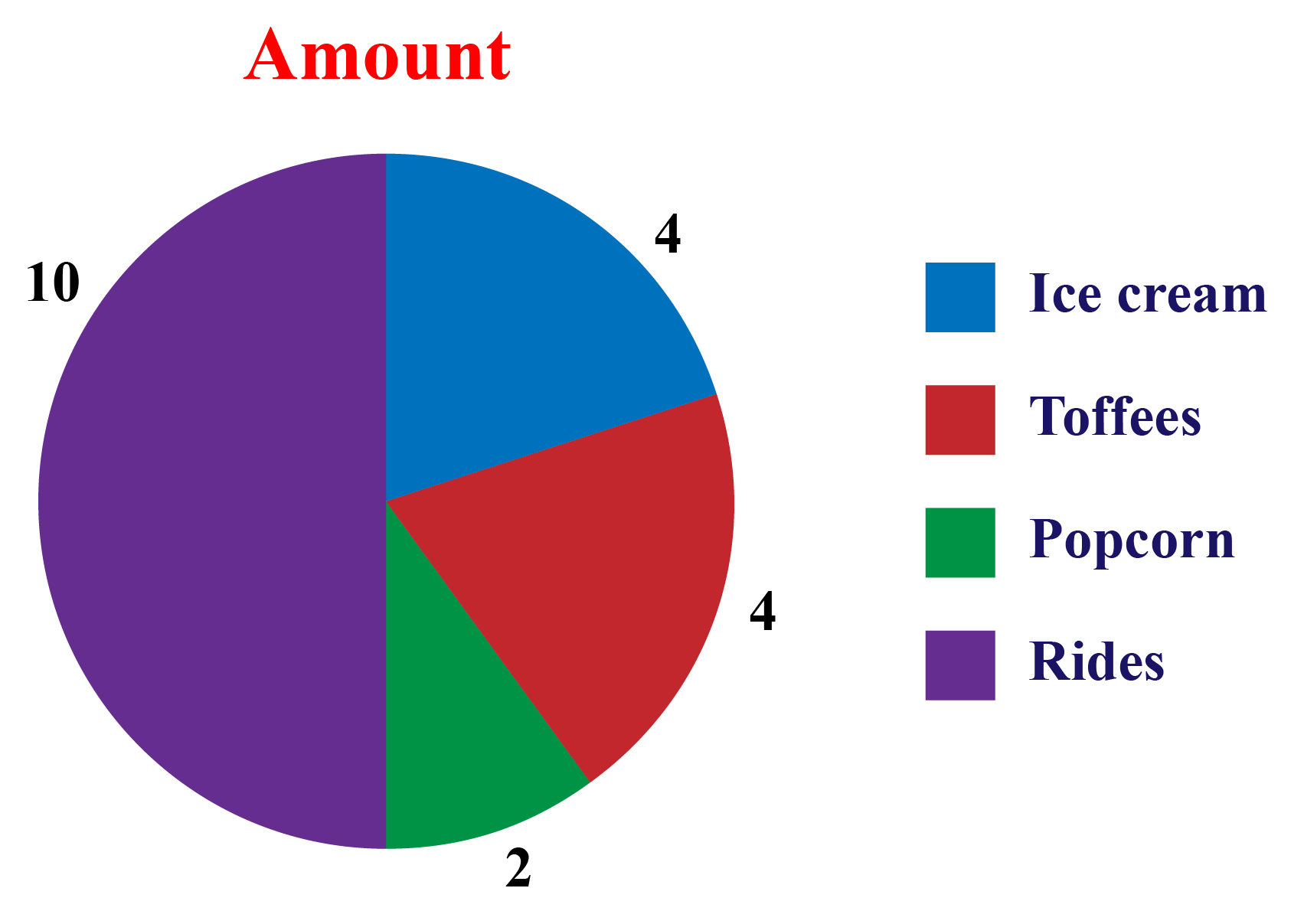The pie chart above shows the amount spent by Ana at the funfair.

The indicated color shows the amount spent on each category.

$$\text{The total value of the data is 20}$$.

The amount spent on each category is interpreted as follows.

Ice Cream Toffees Popcorn Rides
4 4 2 10

To convert this into percentage, we apply this calculation into the pie chart formula:

Amount spent on ice creams

\begin{align}&=\dfrac{10}{20} \times 100\\ &= 50 \%\end{align}

Amount spent on toffees

\begin{align}&=\dfrac{4}{20} \times 100\\ &= 20 \%\end{align}

Amount spent on pop corn

\begin{align}&=\dfrac{2}{20} \times 100\\ &= 10 \%\end{align}

Amount spent on ride

\begin{align}&=\dfrac{4}{20} \times 100\\ &= 20 \%\end{align}

To convert this into pie chart percentage, we apply this calculation:

 $$(\text{Frequency} \div \text{Total Frequency})\times 100$$

We know that a circle subtends an angle of $$360^\circ$$.

Hence the total of all the data is equal to $$360^\circ$$.

To convert this into degrees, we apply this calculation:

 (Given Data $$\div$$ Total value of Data) $$\times 360 ^\circ$$

## What Is a Pie Chart Used for?

Whenever some data has to be represented visually as a fractional part of a whole, we use pie charts.

Pie charts are used to display the percentage of the total.

A pie chart is used to perfectly compare the areas and see why one is smaller/greater than the other.

Therefore, when we are dealing with a limited number of buckets and discrete data sets, it’s better to use a pie chart.

Try creating the pie chart by giving any discrete data in the simulation below.

## How Do You Calculate Pie Charts?

Given a pie chart, we get the worth of each slice by measuring the angle.

### Method

To calculate the percentage each slice is worth, measure the angle of each slice.

Divide this by $$360$$ then multiply it by $$100$$.

### Pie Chart Example

This pie chart recommends a low-carb diet on a day.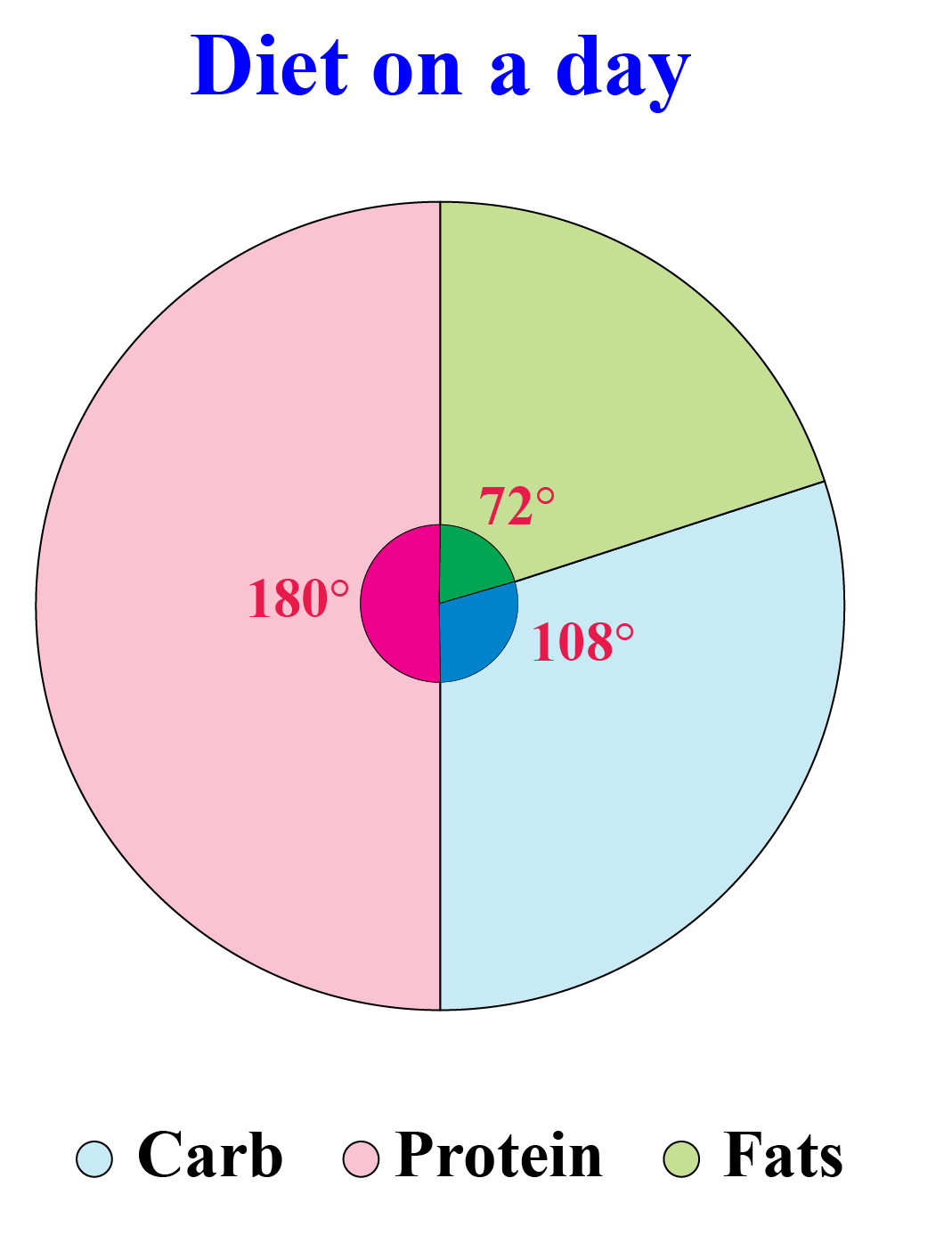We measure the angles of each slice.

We get that "Protein" measures $$180 ^\circ$$ , "Carb" measures $$108^\circ$$ and "Fats" measures $$72^\circ$$.

To find the percentage, we divide each angle by 360 and multiply by 100.

$\text{Protein} = \dfrac{180}{360} \times 100 =50 \%$

$\text{Carb} = \dfrac{108}{360} \times 100 =30 \%$

$\text{Fats} = \dfrac{72}{360} \times 100 = 20 \%$

## How Do You Read Pie Charts?

### Explanation

To read a pie chart or to interpret a pie chart, we see if the given chart is given in percentages or without any value.

If it is given in percentages, the conversion is made accordingly and interpreted accordingly.

The pie chart below shows the percentages of types of transportation used by $$500$$ students to come to school.
a) How many students come to school by bicycle?
b) How many students do not walk to school?
c) How many students come to school by bus or in a car?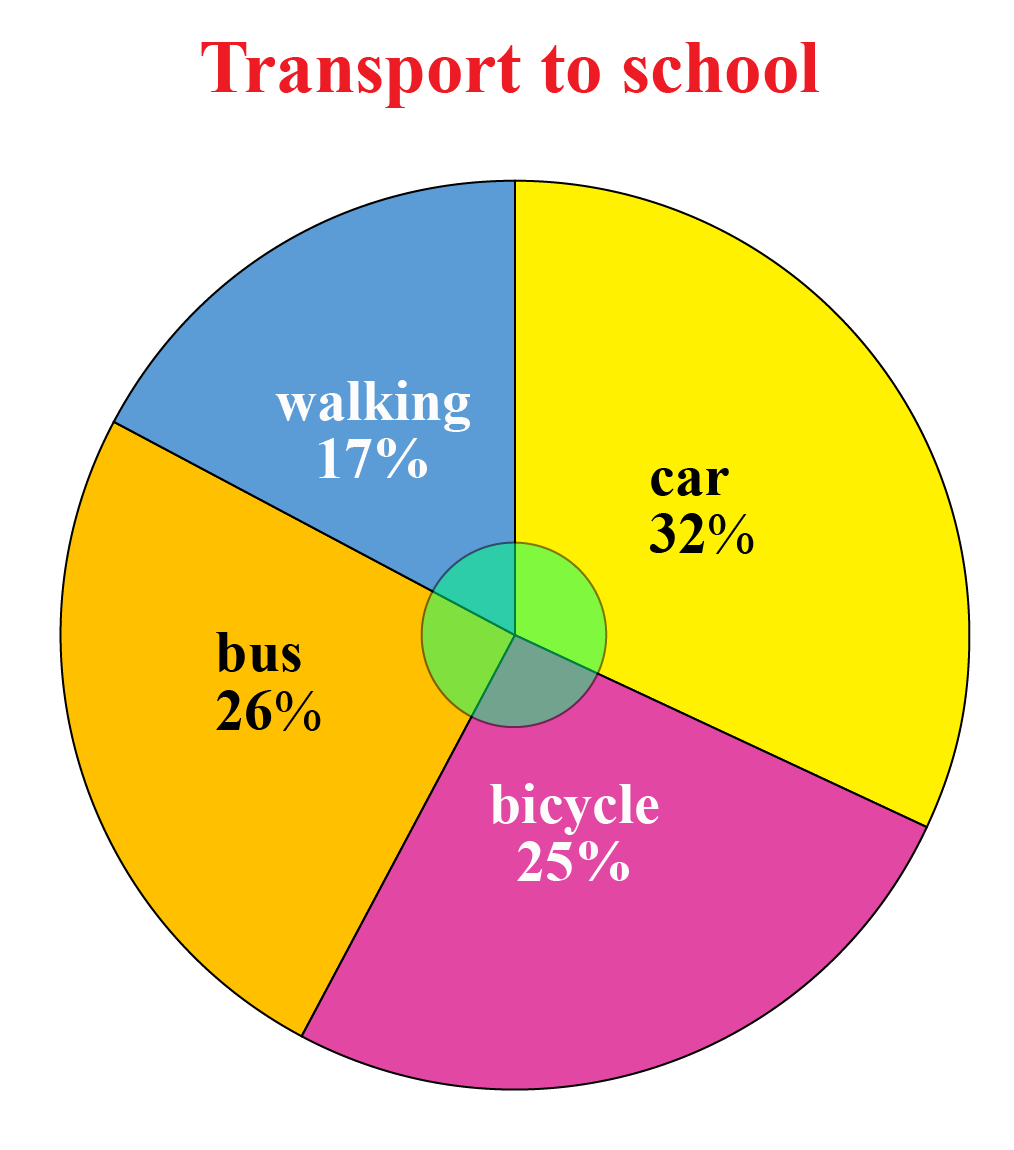a) The students who come by bicycle  \begin{align}&= \dfrac{25}{100} \times 500\\ &= 25 \times 5\\ &= 125\end{align}

b) The students who do not walk to school \begin{align}&= \dfrac{83}{100} \times 500\\ &= 83 \times 5\\ &= 415\end{align}

c)  The students who come by bus or car \begin{align}&=\dfrac{32+26}{100} \times 500\\ &=\dfrac{32+26}{100} \times 500\\ &= 58 \times 5\\&=290\end{align}

## Construction of Pie Charts

Based on the calculations, using the above-mentioned formula, we start collecting the data. Then, we construct the angles in the pie based on the arrived results.

Steps

Step 1: Add the frequency in the table to get the total frequency.
Step 3: Then to know how many degrees for each “pie sector” we need, we will take a full circle of 360° and follow the calculations below:
$(\text{Frequency} \div \text{Total Frequency})\times 360°$
Step 4: Draw a circle and use a protractor to measure the degree of each sector.

Example

Let's construct a pie chart to visually display the favorite fruits of the students in the class based on the frequency table below.

Mango Orange Plum Pineapple  Melon
45 30 15 30 30

The total frequency sums up to 150

Category Formula Degrees
Mango $$\dfrac{45}{150} \times 360$$ $$108^\circ$$
Orange $$\dfrac{30}{150} \times 360$$ $$72^\circ$$
Plum $$\dfrac{15}{150} \times 360$$ $$36^\circ$$
Pineapple $$\dfrac{30}{150} \times 360$$ $$72^\circ$$
Melon $$\dfrac{30}{150} \times 360$$ $$72^\circ$$

Draw a circle and draw the radius.

With the radius as the base, construct $$108^\circ$$ using a protractor.

Subsequently, construct all the other sectors with their respective angles.

Thus we get the pie chart as follows.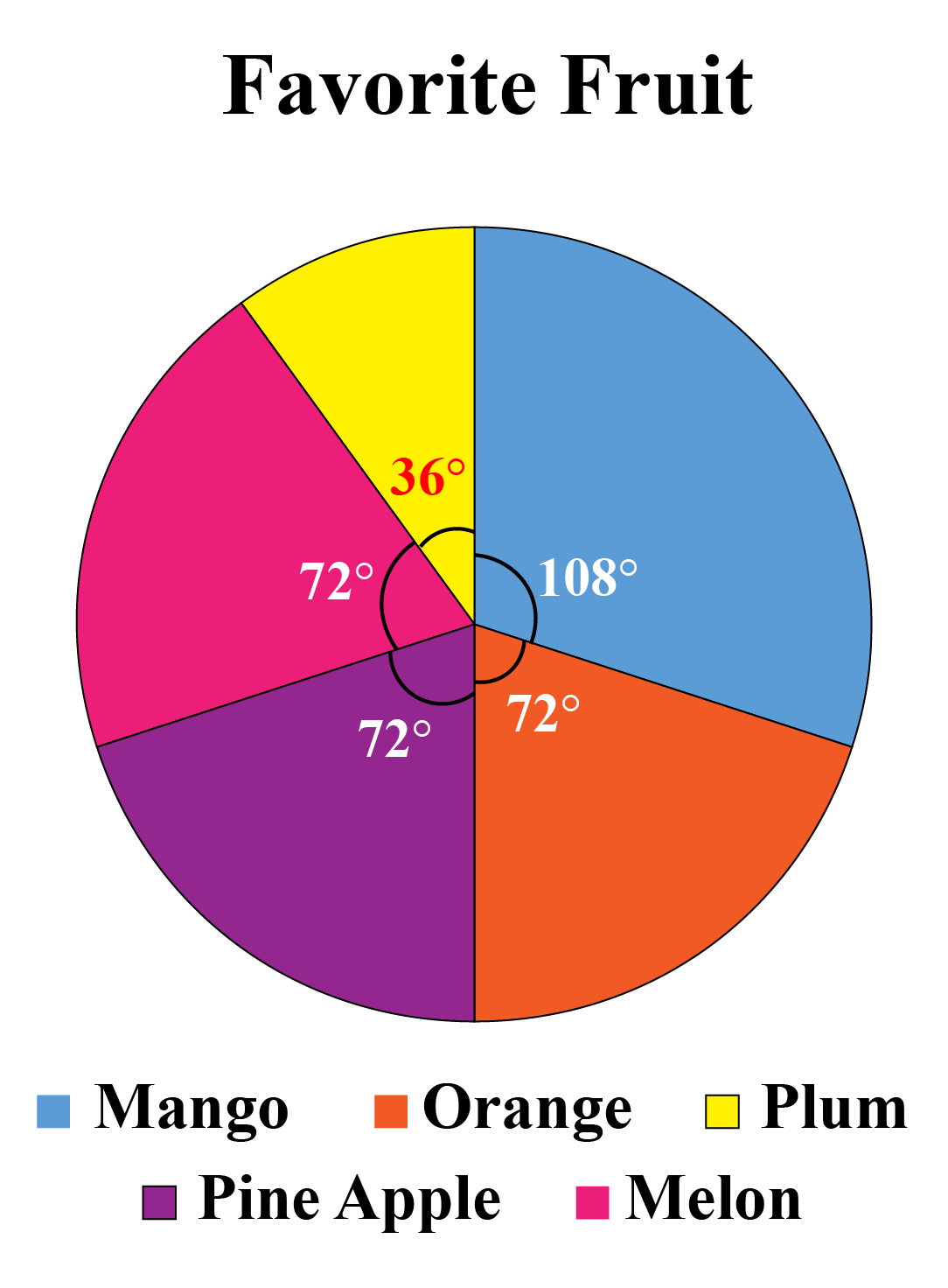## Solved Examples

 Example 1

The following chart shows the activities of Diana in a week.

a) Calculate the central angle subtended at sleeping.

b) Find the portion spent at school.

c) Calculate the time spent on TV per day.

d) Find the portion spent in playing or sleeping.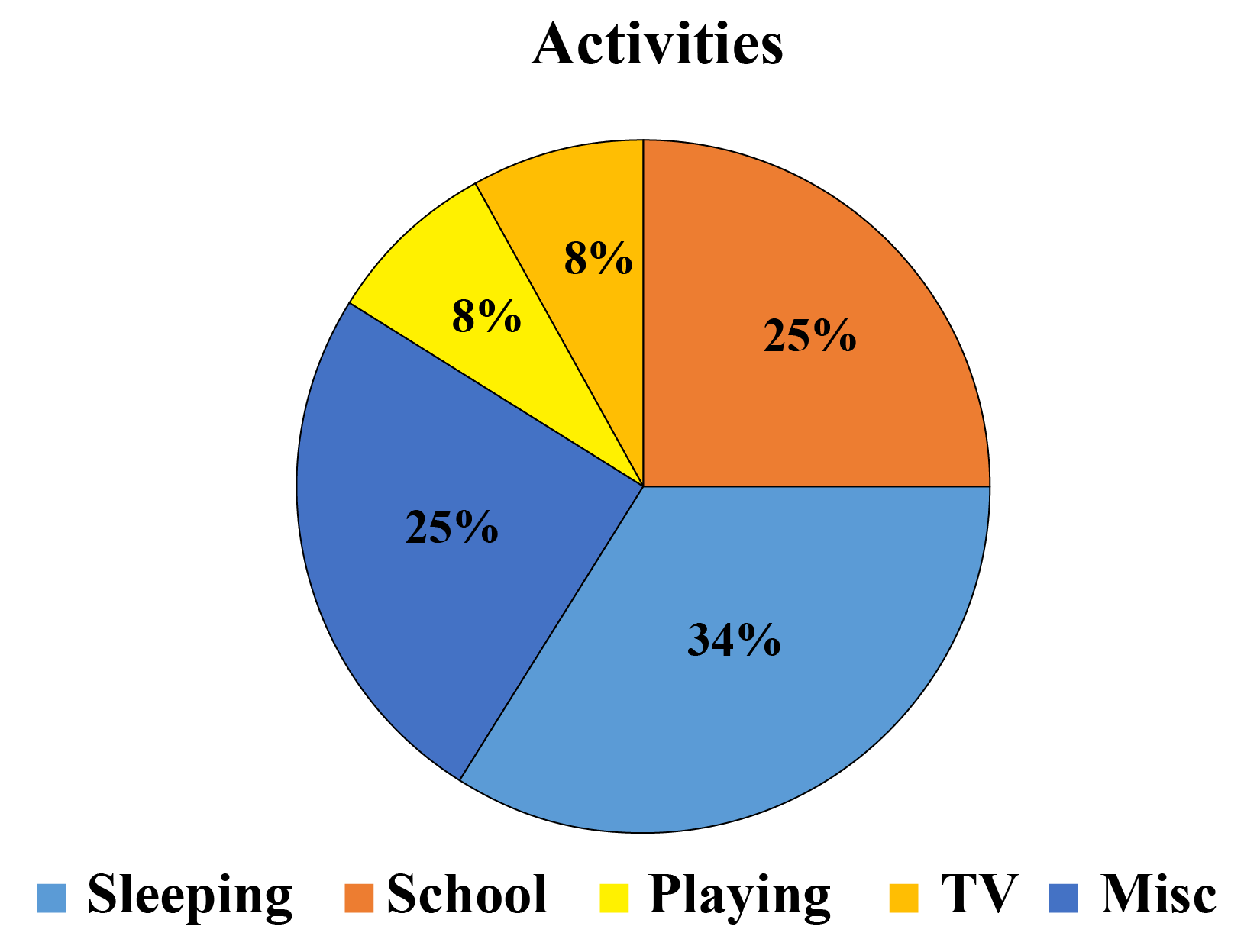Solution

a) Time spent on sleeping \begin{align} &= \dfrac{34}{100}\\&= \dfrac{34}{100} \times 360\\ &=122.4 ^\circ\end{align}

 $$\therefore$$central angle at sleeping = 122.4 $$^\circ$$​​​​

b) Time at school \begin{align}&= \dfrac{25}{100}\\&=\dfrac{1}{4}\end{align}

 $$\therefore$$ portion spent at school = $$\dfrac{1}{4}$$

c) Time spent on TV per day \begin{align}&= \dfrac{8}{100} \times 24\\ &=1.92\text{ hours}\\ &= 1\text{ hour} 55\text{ minutes}\end{align}

 $$\therefore$$ time spent on TV per day = 1 hour 55 minutes

d) Portion spent on playing or sleeping\begin{align}&=\dfrac{8}{100} + \dfrac{34}{100}\\&=\dfrac{42}{100}\\&=\dfrac{21}{50}\end{align}

 $$\therefore$$ portion spent on playing or sleeping= $$\dfrac{21}{50}$$
 Example 2

The pie chart shows the favorite subjects of students in a class.

Using the information given in the pie chart, determine the percentage of students who chose English.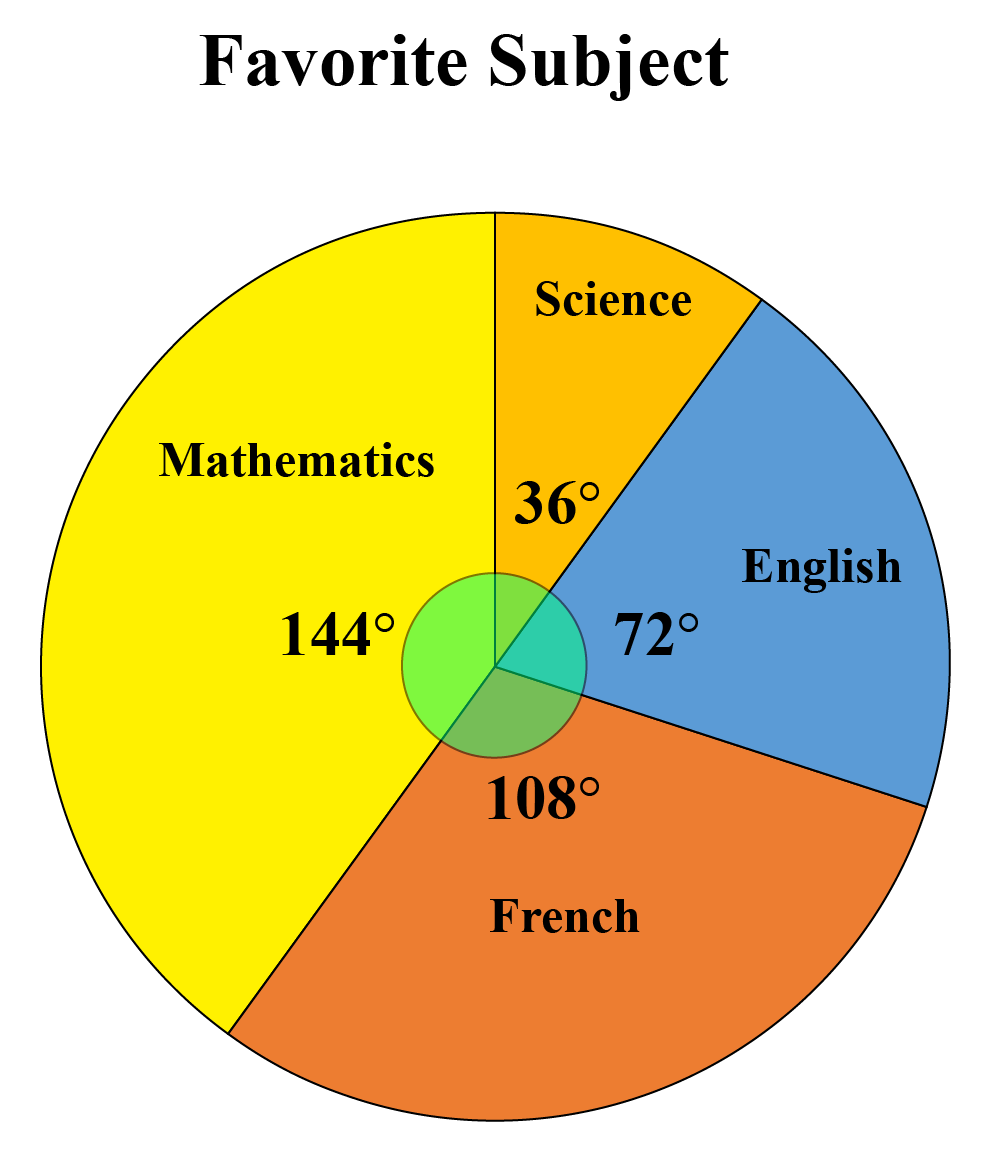Solution

We know that $$144^\circ +36^\circ+72^\circ+108^\circ= 360^\circ$$

The percentage of students who chose English \begin{align}&=\dfrac{72}{360}\times 100\\&=20\end{align}

 $$\therefore$$ The percentage of students who chose English = 20
 Example 3

Using the information given in the pie chart, determine the number of students who chose Mathematics, if the class strength is 40.Solution

We know that $$144 ^\circ+36^\circ+72^\circ+108^\circ= 360^\circ$$

The percentage of students who chose Mathematics in a class of 40 \begin{align} &=\dfrac{144}{360}\times 40\\&= 16\end{align}

 $$\therefore$$ The number of students who chose Mathematics in a class of $$40$$= $$16$$

## Interactive Questions

Here are a few activities for you to practice. Select/Type your answer and click the "Check Answer" button to see the result.Challenging Questions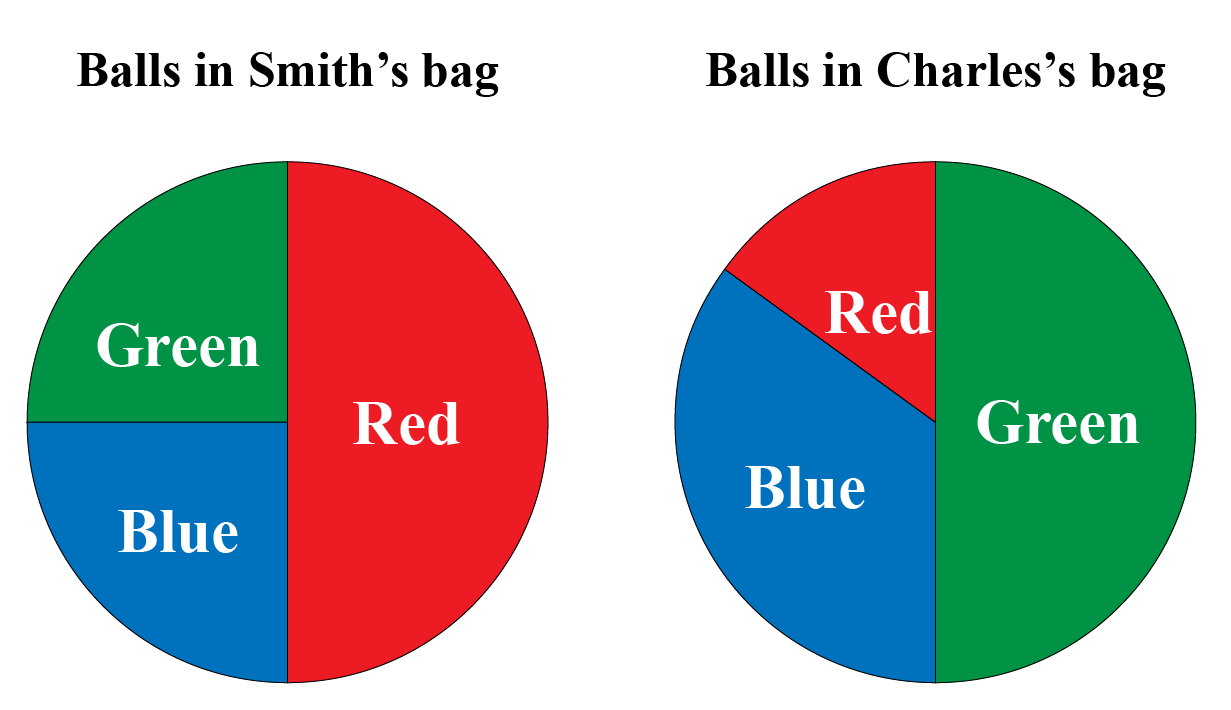1. If each picks one ball from their own bag, who is more likely to get the red ball?
2. If Smith has 12 red balls, how many balls are there altogether in his bag?

## Let's Summarize

We hope you enjoyed learning about Pie Charts with the simulations and practice questions. Now, you will be able to easily solve problems on an explanation of the pie chart, pie graph definition, pie chart examples, and pie chart percentage.

At Cuemath, our team of math experts is dedicated to making learning fun for our favorite readers, the students!

Through an interactive and engaging learning-teaching-learning approach, the teachers explore all angles of a topic.

Be it worksheets, online classes, doubt sessions, or any other form of relation, it’s the logical thinking and smart learning approach that we, at Cuemath, believe in.

## 1. Should a pie chart be equal to 100?

The total value of the pie chart should be equal to 100. The slices of the pie chart are proportional to the whole.

## 2. What is a pie chart maker?

Pie-chart makers are the online tools that help us create circle charts for the purposes of business.

The data is keyed in, the pie chart is customized by choosing the template, color  etc. and then created at the user's convenience.

## 3. Give pie chart examples with data.

###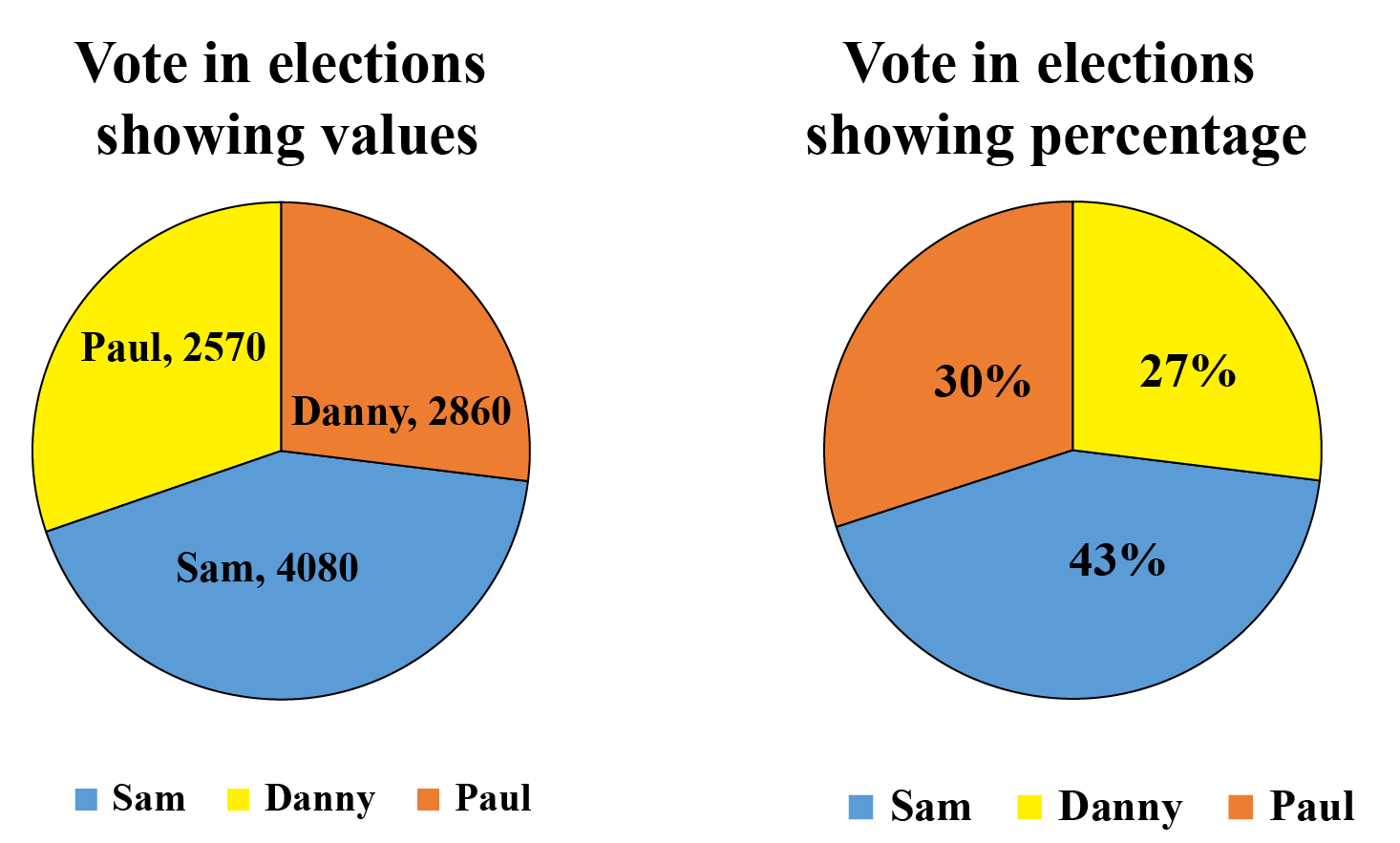The pie chart is read in two ways:

The percentage is given and the values have to be calculated.

Values are given and the percentage has to be determined.

## 4. How to change pie chart percentage to numbers?

If the pie chart displays the percentage, the conversion into values is done using the formula $$(\text{given data} \div {100})\\ \times \text {total frequency of the data}$$.

More Important Topics
Numbers
Algebra
Geometry
Measurement
Money
Data
Trigonometry
Calculus